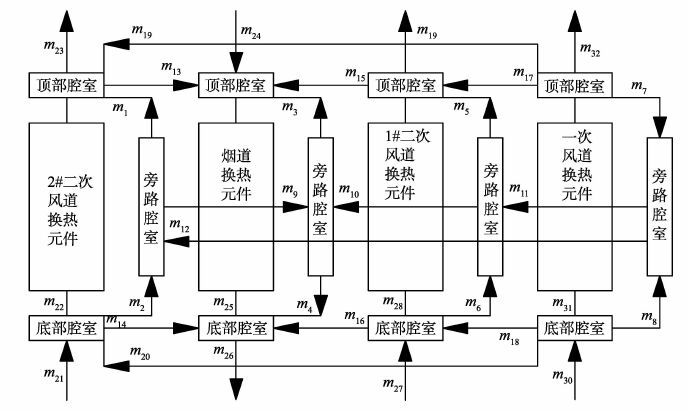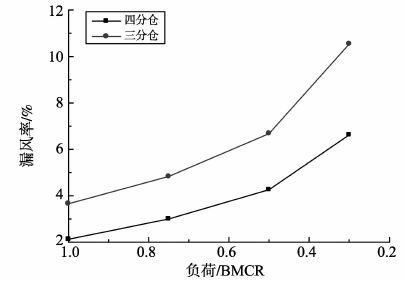﻿ 四分仓回转空预器热力模型与漏风模型耦合计算文章快速检索 高级检索
 山东大学学报(工学版)2016, Vol. 46Issue (5): 126-131  DOI: 10.6040/j.issn.1672-3961.0.2016.0360

### 引用本文CHU Da, SHI Yuetao, WANG Xiaojuan. Coupling calculation between thermal model and air leakage model of quad-sectional rotary air preheater[J]. JOURNAL OF SHANDONG UNIVERSITY (ENGINEERING SCIENCE), 2016, 46(5): 126-131. DOI: 10.6040/j.issn.1672-3961.0.2016.036.### 文章历史

Coupling calculation between thermal model and air leakage model of quad-sectional rotary air preheater
CHU Da, SHI Yuetao, WANG Xiaojuan
School of Energy and Power Engineering, Shandong University, Jinan 250061, Shandong, China
Abstract: In order to analyze the heat transfer performance and low-temperature corrosion resistance property of quad-sectional rotary air preheater, the thermal and air-leakage calculation model were built and a concept named low-temperature area rate was proposed. With the air leakage ignored, thermal model was solved by numerical finite difference method based on control equations of rotary air preheater and temperature distribution was acquired. The energy and mass equations of air leakage were solved according to the results of thermal calculation and air leakage rate was obtained. Then iterative calculation of these two models was carried out in accordance with inlet and outlet parameters. The results showed that the difference between the calculated value and the designed value was less than 5％. Air leakage of quad-sectional air preheater was much less than that of tri-sectional one with the same heat transfer area. The temperature distributions of fluids in different channels were continuous and consistent with matrix. The low-temperature area rate was reduced with the decline of inlet gas flow along the direction of rotary, which helped to avoid low-temperature corrosion.
Key words: quad-sectional rotary air preheater    thermal model    air leakage model    low-temperature corrosion    low-temperature area rate
0 引言

1 计算模型 1.1 物理模型图 1 物理模型示意图 Figure 1 Schematic diagram of physical model

1.2 求解热力模型

 $\begin{array}{*{35}{l}} {{w}_{g}}{{\rho }_{g}}{{c}_{p}}\left( 1-{{\varphi }_{z}} \right)\frac{\partial {{T}_{g}}}{\partial z}+\omega {{\sigma }_{m}}{{\rho }_{m}}{{c}_{m}}\frac{\partial {{T}_{m}}}{\partial \theta }- \\ \lambda \left[ {{\varphi }_{z}}\frac{{{\partial }^{2}}{{T}_{m}}}{\partial {{z}^{2}}}+{{\varphi }_{\theta }}\frac{1{{\partial }^{2}}{{T}_{m}}}{{{r}^{2}}\partial {{\theta }^{2}}}+{{\varphi }_{r}}\left( \frac{1\partial {{T}_{m}}}{r\partial r}+\frac{{{\partial }^{2}}{{T}_{m}}}{\partial {{r}^{2}}} \right) \right]=0, \text{ }\left( 1 \right) \\ \end{array}$ (1)
 $-{{w}_{g}}{{\rho }_{g}}{{c}_{p}}(1-{{\varphi }_{z}})\frac{\partial {{T}_{g}}}{\partial z}-\alpha \beta ({{T}_{g}}-{{T}_{m}})=0, \text{ }$ (2)

1.3 漏风模型

 $m={{C}_{d}}{{A}_{i}}Y\sqrt{2{{\rho }_{i}}\Delta {{p}_{i}}},$ (3)

1.4 漏风模型与热力模型耦合图 2 计算流程图 Figure 2 Calculation flow chart
2 实际算例及结果分析 2.1 原始数据表 1 已知参数 Table 1 Known parameters表 2 网格独立性验证 Table 2 Mesh independence verification
2.2 计算结果分析图 3 流体温度分布图 Figure 3 Fluid temperature field图 4 换热元件温度分布图 Figure 4 Matrix temperature field图 5 低温区域比与函数斜率关系图 Figure 5 Relation graph of low-temperature area and funtion dope表 3 各处漏风量 Table 3 Air leakage mass flow kg/s图 6 不同负荷下三分仓与四分仓空预器漏风率对比 Figure 6 The comparison of air leakage rate between tri-sectional and quad-sectional air preheater under different load
3 结论

  陈昌贤, 孙奉仲, 李飞, 等. 四分仓回转式空气预热器热力计算方法[J]. 山东大学学报(工学版) , 2014, 44 (4) : 60-65 CHEN Changxian, SUN Fengzhong, LI Fei, et al. Thermal calculation method for quad-sectional regenerative air preheater[J]. Journal of Shandong University (Engineering Science) , 2014, 44 (4) : 60-65  周俊虎, 杨卫娟, 靳彦涛, 等. 三分仓空气预热器热力计算的研究[J]. 动力工程 , 2003, 23 (6) : 2810-2813 ZHOU Junhu, YANG Weijuan, JIN Yantao, et al. Research of tri-sectional air preheater thermal calculation[J]. Power Engineering , 2003, 23 (6) : 2810-2813  冷伟, 王渡. 一种改进的回转式空气预热器热力计算方法[J]. 动力工程 , 2005, 25 (3) : 392-395 LENG Wei, WANG Du. An improved way for thermal calculation of rotary air preheater[J]. Power Engineering , 2005, 25 (3) : 392-395  郑凯, 周克毅, 黄军林, 等. 一种改进的三分仓空气预热器热力计算方法[J]. 动力工程学报 , 2012, 32 (1) : 31-35 ZHENG Kai, ZHOU Keyi, HUANG Junlin, et al. An improved thermal calculation method for tri-sectional rotary air preheaters[J]. Journal of Chinese Society of Power Engineeryin , 2012, 32 (1) : 31-35  SKIEPKO T, SHAH R K. A comparison of rotary regenerator theory and experimental results for an air preheater for a thermal power plant[J]. Experimental Thermal and Fluid Science , 2004, 28 (28) : 257-264  张启, 王谦, 王恩禄, 等. 基于有限差分的二分仓回转式空气预热器热力计算[J]. 锅炉技术 , 2009, 40 (4) : 25-29 ZHANG Qi, WANG Qian, WANG Enlu, et al. Bisector air preheater performance evaluation based on numerical finite difference method[J]. Boiler Technology , 2009, 40 (4) : 25-29  刘福国, 周新刚. 考虑轴向导热的三分仓回转预热器传热模型及验证[J]. 机械工程学报 , 2010, 46 (22) : 144-150 LIU Fuguo, ZHOU Xingang. Heat transfer model of tri-section rotary air preheater and experimental verification[J]. Journal of Mechanical Engineering , 2010, 46 (22) : 144-150 DOI:10.3901/JME.2010.22.144  HEIDARI-KAYDAN A, HAJIDAVALLOO E. Three-dimensional simulation of rotary air preheater in steam power plant[J]. Applied Thermal Engineering , 2014 (73) : 399-407  冷伟, 陈道轮, 张志伦, 等. 基于解析方法的回转式空气预热器换热计算[J]. 中国电机工学报 , 2005, 25 (3) : 141-146 LENG Wei, CHEN Daolun, ZHANG Zhilun, et al. Heat exchange calculation of regenerative air preheater with analytical method[J]. Proceeding of the CSEE , 2005, 25 (3) : 141-146  SKIEPKO T. Experimental results concerning seal clearances in some rotary heat exchangers[J]. Heat Recovery Systems , 1988, 8 (6) : 577-871 DOI:10.1016/0890-4332(88)90017-8  ZENG M, DU L, LIAO D, et al. Investigation on pressure drop and heat transfer performances of plate-fin iron air preheater unit with experimental and genetic algorithm methods[J]. Applied Energy , 2012, 92 (4) : 725-732  WANG L, DENG L, TANG C, et al. Thermal deformation prediction based on the temperapture distribution of the rotor in rotary air-preheater[J]. Applied Thermal Egineering , 2015, 90 : 478-488 DOI:10.1016/j.applthermaleng.2015.07.021  CAI M, HUI S, WANG X, et al. A study on the direct leakage of rotary air preheater with multiple seals[J]. Applied Thermal Engineering , 2013, 59 (1-2) : 576-586 DOI:10.1016/j.applthermaleng.2013.05.049  JESTIN L, FULS W, PRONOBIS M. A numerical study of air preheater leakage[J]. Energy , 2015, 92 : 37-44  SHAH R K, SKIEPKO T. Influence of leakage distribution on the thermal performance of a rotary regenerator[J]. Applied Thermal Engineering , 1999, 19 : 685-705 DOI:10.1016/S1359-4311(98)00087-8